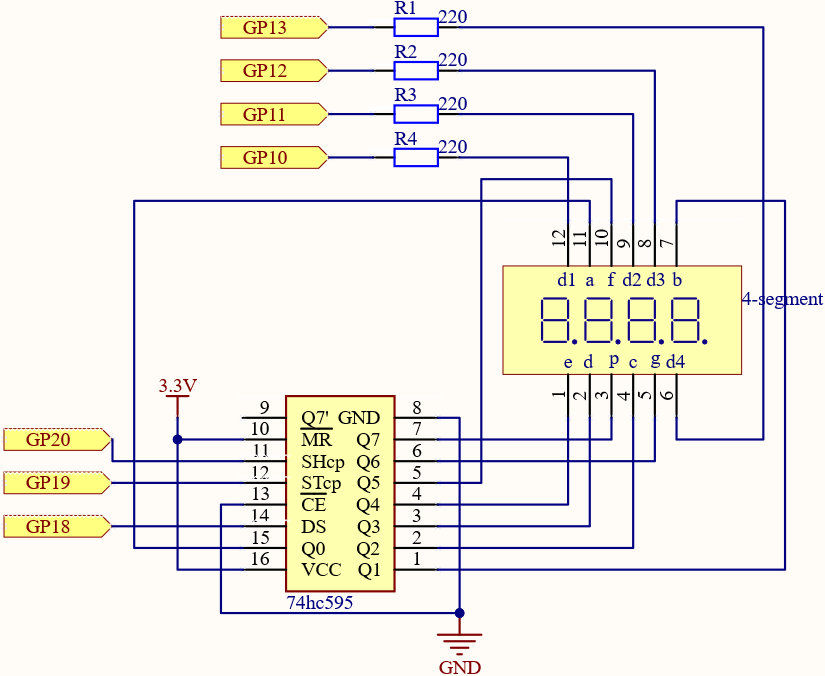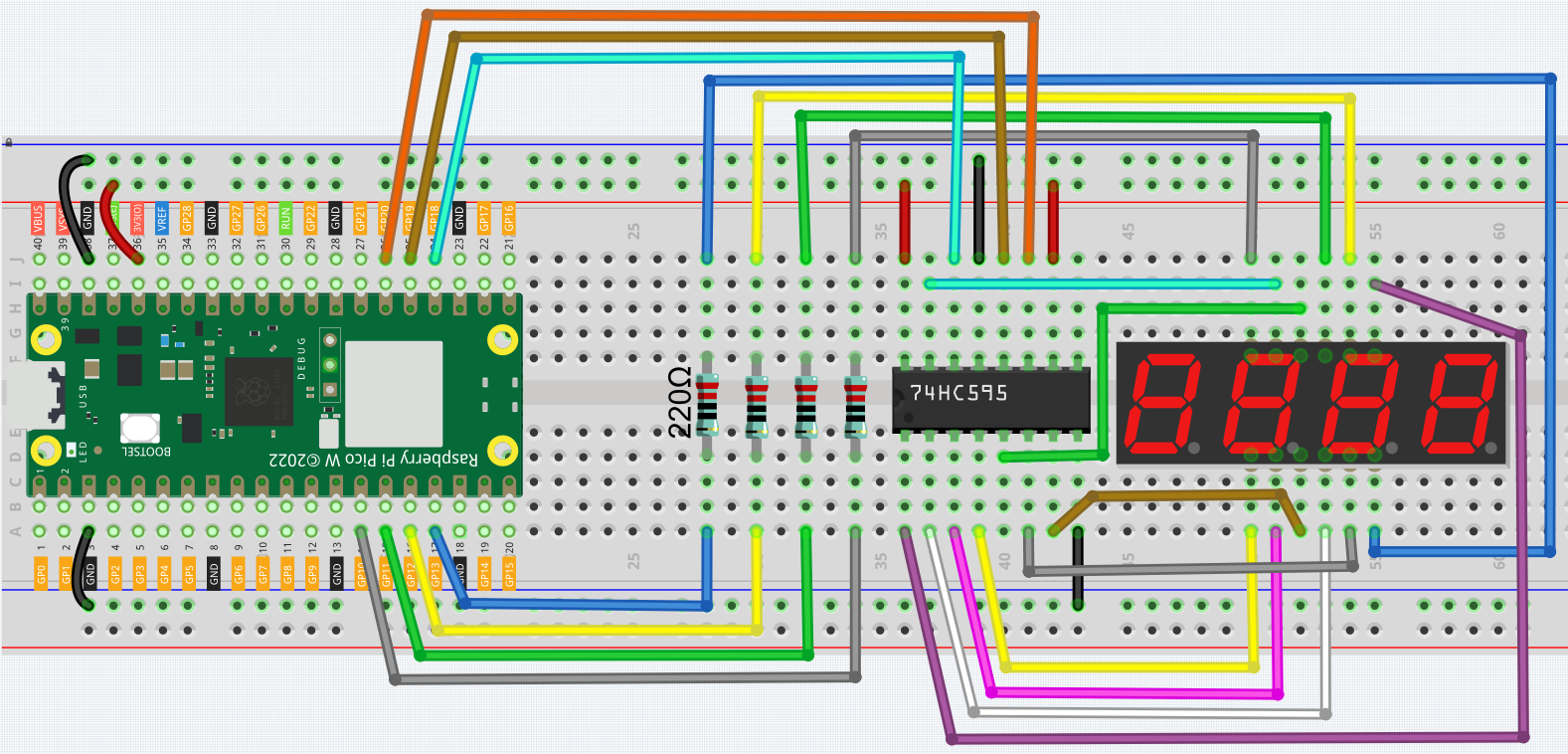# 5.3 Time Counter¶

4-digit 7-segment display consists of four 7-segment displays working together.

The 4-digtal 7-segment display works independently. It uses the principle of human visual persistence to quickly display the characters of each 7-segment in a loop to form continuous strings.

For example, when “1234” is displayed on the display, “1” is displayed on the first 7-segment, and “234” is not displayed. After a period of time, the second 7-segment shows “2”, the 1st 3th 4th of 7-segment does not show, and so on, the four digital display show in turn. This process is very short (typically 5ms), and because of the optical afterglow effect and the principle of visual residue, we can see four characters at the same time.

SchematicHere the wiring principle is basically the same as 5.1 Microchip - 74HC595, the only difference is that Q0-Q7 are connected to the a ~ g pins of the 4-digit 7-segment display.

Then G10 ~ G13 will select which 7-segment display to work.

WiringCode

Note

• Open the `5.3_time_counter.py` file under the path of `kepler-kit-main/micropython` or copy this code into Thonny, then click “Run Current Script” or simply press F5 to run it.

• Don’t forget to click on the “MicroPython (Raspberry Pi Pico)” interpreter in the bottom right corner.

• For detailed tutorials, please refer to Open and Run Code Directly.

```import machine
import time

SEGCODE = [0x3f,0x06,0x5b,0x4f,0x66,0x6d,0x7d,0x07,0x7f,0x6f]

sdi = machine.Pin(18,machine.Pin.OUT)
rclk = machine.Pin(19,machine.Pin.OUT)
srclk = machine.Pin(20,machine.Pin.OUT)

placePin = []
pin = [10,13,12,11]
for i in range(4):
placePin.append(None)
placePin[i] = machine.Pin(pin[i], machine.Pin.OUT)

timerStart=time.ticks_ms()

def timer1():
return int((time.ticks_ms()-timerStart)/1000)

def pickDigit(digit):
for i in range(4):
placePin[i].value(1)
placePin[digit].value(0)

def clearDisplay():
hc595_shift(0x00)

def hc595_shift(dat):
rclk.low()
time.sleep_us(200)
for bit in range(7, -1, -1):
srclk.low()
time.sleep_us(200)
value = 1 & (dat >> bit)
sdi.value(value)
time.sleep_us(200)
srclk.high()
time.sleep_us(200)
time.sleep_us(200)
rclk.high()
time.sleep_us(200)

while True:
count = timer1()
#print(count)

pickDigit(0)
hc595_shift(SEGCODE[count%10])

pickDigit(1)
hc595_shift(SEGCODE[count%100//10])

pickDigit(2)
hc595_shift(SEGCODE[count%1000//100])

pickDigit(3)
hc595_shift(SEGCODE[count%10000//1000])
```

After the program is run, you will see the 4-digit 7-segment display become a counter and the number increases by 1 per second.

How it works?

Writing signals to each 7-segment display is done in the same way as 5.2 Number Display, using the `hc595_shift()` function. The core point of the 4-digit 7-segment display is to selectively activate each 7-segment display. The code associated with this is as follows.

```placePin = []
pin = [13,12,11,10]
for i in range(4):
placePin.append(None)
placePin[i] = machine.Pin(pin[i], machine.Pin.OUT)

def pickDigit(digit):
for i in range(4):
placePin[i].value(1)
placePin[digit].value(0)

while True:

hc595_shift(SEGCODE[count%10])
pickDigit(0)

hc595_shift(SEGCODE[count%100//10])
pickDigit(1)

hc595_shift(SEGCODE[count%1000//100])
pickDigit(2)

hc595_shift(SEGCODE[count%10000//1000])
pickDigit(3)
```

Here, four pins (GP10, GP11, GP12, GP13) are used to control each bit of the 4-digit 7-segment display individually. When the state of these pins is `0`, the corresponding 7-segment display is active; when the state is `1`, the opposite is true.

Here the `pickDigit(digit)` function is used to unable all four digits and then enable a particular digit individually. After that, `hc595_shift()` is used to write the corresponding 8 bits code for the 7-segment display.

The 4-digit 7-segment display needs to be continuously activated in turn so that we can see it display four digits, which means that the main program cannot easily add code that would affect the timing. However, we need to add a timing function to this example, and if we add a `sleep(1)`, we will know that it has four digits. we will see through the illusion of 4-digit 7-segment display working at the same time, exposing the fact that only one 7-segment display is illuminated at a time. Then, using the `time.ticks_ms()` function in the `time` library is an excellent way to do this.

```import time

timerStart=time.ticks_ms()

def timer1():
return int((time.ticks_ms()-timerStart)/1000)

while True:
count = timer1()
```

The `time.ticks_ms()` function gets a (non-explicit) time, and we record the first time value we get as `timerStart`. Subsequently, when the time is needed, the `time.ticks_ms()` function is called again, and the value is subtracted from `timerStart` to get how long the program has been running (in milliseconds).

Finally, convert and output this time value to the 4-digit 7-segment display and you’re done.